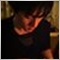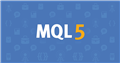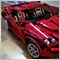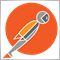# mt5.order_send() returns None22

Thank you in advance for helping me!

I'm coding automatic trading system in python.

I'd like to get positions and close them automatically.

However, mt5.order_send() returns None(not any errors but 'None'!) if request['position'] is input from pd.Series data.

When I directly input position id code, the function works.

the code is below:

*mt5_initialize() is user function that calls mt5.initialize()

*tickets[i] is the position id (int32).

```def close_positions(tickets, symbol, lot):
symbol = tickets.name
for i in range(len(tickets)):
mt5_initialize()
price = mt5.symbol_info_tick(symbol).bid
deviation = 20
position_id = tickets[i]
request = {
'symbol': symbol,
'volume': lot,
'type': mt5.ORDER_TYPE_SELL,
'position': position_id,
'price': price,
'deviation': deviation,
'comment': 'python script close',
'type_time': mt5.ORDER_TIME_GTC,
'type_filling': mt5.ORDER_FILLING_FOK,
}
request['position'] = tickets[i]
result = mt5.order_send(request)
print(result)
print('3. close position #{}: sell {} {} lots at {} with deviation={} points'.
format(position_id, symbol, lot, price, deviation))
print('4. order_send failed, because:', error_desc[result.retcode])
print('   result', result)
else:
print('4. position #{} closed, {}'.format(position_id, result))
mt5.shutdown()
print('close positions of', symbol)
return None
```Documentation on MQL5: Constants, Enumerations and Structures / Trade Constants / Trade Operation Types
• www.mql5.com
Trading is done by sending orders to open positions using the OrderSend() function, as well as to place, modify or delete pending orders. Each trade order refers to the type of the requested operation. Trading operations are described in the ENUM_TRADE_REQUEST_ACTIONS enumeration...1742

However, mt5.order_send() returns None(not any errors but 'None'!) if request['position'] is input from pd.Series data.

When I directly input position id code, the function works.

This function is fine (apart from the redundant but harmless "request['position'] = tickets[i]").

Are you able to show the lines that calls close_positions()? Include parts that fill up "tickets"...22

Seng Joo Thio:

This function is fine (apart from the redundant but harmless "request['position'] = tickets[i]").

Are you able to show the lines that calls close_positions()? Include parts that fill up "tickets"...

Hi Seng, thank you for cooperation!

This is the logic that get tickets:

```# get tickets of open positions
def get_tickets(symbol):
mt5_initialize()
positions = mt5.positions_get(symbol=symbol)
tickets = pd.DataFrame(index=range(len(positions)), columns=['ticket', 'volume'])
for i in range(len(positions)):
tickets.loc[i, 'ticket'] = positions[i].ticket
tickets.loc[i, 'volume'] = positions[i].volume
mt5.shutdown()
tickets['ticket'] = tickets['ticket'].astype('int')
tickets['volume'] = tickets['volume'].astype('float')
tickets['symbol'] = symbol
return tickets

# get single open position
def get_position(symbol):
mt5_initialize()
positions = mt5.positions_get(symbol=symbol)
mt5.shutdown()
net_position = 0
net_profit = 0
if len(positions) >= 1:
for i in range(len(positions)):
if positions[i].type == 0:
sign = 1
elif positions[i].type == 1:
sign = -1
net_position += positions[i].volume * sign
net_profit += (positions[i].price_current - positions[i].price_open) * positions[i].volume * sign
net_amount = net_position * positions.price_current
else:
net_amount = 0
net_profit = 0
return net_amount, net_profit

```

Using these functions, I execute this way:

tickets = get_tickets('BTCJPY')

close_positions(tickets)2466

Pandas is good for working with large sets of data, but it's inefficient to setup and tear down. It's also unnecessary since mt5 is returning pure python objects, so it's better to work with pure python for what you're doing. In addition, you should only setup and tear-down the mt5 module only once in your program. Doing it inside of each loop iteration is absolutely going to kill your script performance. Here's an example:

```import MetaTrader5 as mt5

def raw_order(**kwargs):
return mt5.order_send(kwargs)

def main():
for pos in mt5.positions_get():
info = mt5.symbol_info_tick(pos.symbol)
result = raw_order(
type=order_type,
symbol=pos.symbol,
volume=pos.volume,
position=pos.ticket,
price=price,
comment="close all",
)
print(result)

for ord in mt5.orders_get():
print(result)

if __name__ == "__main__":
if mt5.initialize():
main()
mt5.shutdown()
```22

nicholi shen:

Pandas is good for working with large sets of data, but it's inefficient to setup and tear down. It's also unnecessary since mt5 is returning pure python objects, so it's better to work with pure python for what you're doing. In addition, you should only setup and tear-down the mt5 module only once in your program. Doing it inside of each loop iteration is absolutely going to kill your script performance. Here's an example:

Hi nicholi,

Thank you for the sample code.

I'd like to close existing open positions (= netting), but the method that you showed seems to order opposite new positions (=hedging).

Is there any way to close positions via python API?2466

Hi nicholi,

Thank you for the sample code.

I'd like to close existing open positions (= netting), but the method that you showed seems to order opposite new positions (=hedging).

Is there any way to close positions via python API?

That's how you close either with MT5. In fact, there is even a Close function included in the MetaTrader5 module so your code could be greatly reduced and refactored to something like this.

```def open_position_symbols():
return tuple(set(p.symbol for p in mt5.positions_get()))

def net_position(symbol):
return sum(
for p in mt5.positions_get(symbol=symbol)
)

def net_profit(symbol):
return sum(p.profit for p in mt5.positions_get(symbol=symbol))

def verbose_status(symbol):
return '{} - Net position = {}, Net profit = {}'.format(
symbol,
net_position(symbol),
net_profit(symbol)
)

def close_all():
for symbol in open_position_symbols():
print(f'Closing: {verbose_status(symbol)}')
mt5.Close(symbol=symbol)

if __name__ == "__main__":
try:
if mt5.initialize():
close_all()
finally:
mt5.shutdown()
```22

nicholi shen:

That's how you close either with MT5. In fact, there is even a Close function included in the MetaTrader5 module so your code could be greatly reduced and refactored to something like this.

Thank you very much!!

I didn't know mt5.Close() function.

Have a nice weekend!22

Thank you very much!!

I didn't know mt5.Close() function.

Have a nice weekend!

Dear nicholi,

First some times the function works well, but it gets not to work sometimes.

Do you know the reason why?2466

No idea why but you really shouldn't be closing orders that way on a hedging account anyway. You would first want to flatten the position the reconcile the orders as closed using the closeby feature.

```import MetaTrader5 as mt5

def raw_order(**kwargs):
return mt5.order_send(kwargs)

def open_position_symbols():
symbols = list(set(p.symbol for p in mt5.positions_get()))
symbols = sorted(symbols, key=lambda s: abs(net_position(mt5.positions_get(symbol=s))), reverse=True)
return symbols

def net_position(positions):
return sum(p.volume if p.type == mt5.POSITION_TYPE_BUY else -p.volume for p in positions)

def flatten(symbol):
positions = mt5.positions_get(symbol=symbol)
net_pos = net_position(positions)
if net_pos == 0:
info = mt5.symbol_info_tick(symbol)
order_type, price, comment = (
(mt5.ORDER_TYPE_SELL, info.bid, "flatten long"),
)[net_pos < 0]
result = raw_order(
type=order_type,
symbol=symbol,
volume=abs(net_pos),
price=price,
comment=comment,
)
return result.retcode

def reconcile(symbol):
positions = mt5.positions_get(symbol=symbol)
if not positions:
try:
long = next(p for p in positions if p.type == mt5.POSITION_TYPE_BUY)
short = next(p for p in positions if p.type == mt5.POSITION_TYPE_SELL)
except StopIteration:
result = raw_order(
position=long.ticket,
position_by=short.ticket,
)
return reconcile(symbol)

def close_all():
symbols = open_position_symbols()
for symbol in symbols:
if (res := flatten(symbol)) != mt5.TRADE_RETCODE_DONE:
print(f"CloseAllError: did not flatten {symbol}, error code={res}")
for symbol in symbols:
if (res := reconcile(symbol)) != mt5.TRADE_RETCODE_DONE:
print(f"CloseAllError: could not reconcile {symbol}, error code={res}")

if __name__ == "__main__":
try:
if mt5.initialize():
close_all()
finally:
mt5.shutdown()
```